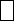Mathematics
Easy

Question

# Find the missing digit in the equation.99 -3 = 16

## 8567Hint:

## The correct answer is: 8

### Let the unknown number be x99-x=1699-16=x99 – 16 = 83The missing digit is 8

It is a problem of linear equations.

### Related Questions to study#### With Turito Foundation.#### Get an Expert Advice From Turito.